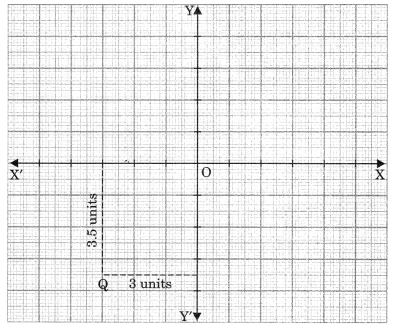# CBSEtips.in

## Saturday 20 February 2021

### CBSE Class 9 Maths - MCQ and Online Tests - Unit 3 - Coordinate Geometry

#### CBSE Class 9 Maths – MCQ and Online Tests – Unit 3 – Coordinate Geometry

Every year CBSE  schools conducts Annual Assessment exams for 6,7,8,9,11th standards. These exams are very competitive to all the students. So our website provides online tests for all the 6,7,8,9,11th standard’s subjects. These tests are also very effective and useful for those who preparing for any competitive exams like Olympiad etc. It can boost their preparation level and confidence level by attempting these chapter wise online tests.

These online tests are based on latest CBSE syllabus. While attempting these our students can identify the weak lessons and continuously practice those lessons for attaining high marks. It also helps to revise the NCERT textbooks thoroughly.

#### CBSE Class 9 Maths – MCQ and Online Tests – Unit 3 – Coordinate Geometry

Question 1.
Mirror image of the point (9, -8) in y-axis is
(a) (-9, -8)
(b) (9, 8)
(c) (-9, 8)
(d) (-8, 9)

Question 2.
The coordinates of the point which lies on y-axis at a distance of 4 units in negative direction of y-axis is
(a) (5, 4)
(b) (4, 0)
(c) (0, -4)
(d) (-4, 0)

Question 3.
If the points A(2, 0), B(-6, 0) and C(3, a – 3) lie on the x-axis, then the value of a is
(a) 0
(b) 2
(c) 3
(d) -6

Question 4.
Which of the following points lies on the negative side of x axis?
(a) (-4, 0)
(b) (3, 2)
(c) (0, -4)
(d) (5, -7)

Question 5.
Which is the example of geometrical line?
(a) Blackboard
(b) Sheet of paper
(c) Meeting place of two walls
(d) Tips of sharp pencil.

Answer: (c) Meeting place of two walls

Question 6.
The Coordinates of point Q are(a) (3, 3.5)
(b) (3.5, 3)
(c) (-3, 3.5)
(d) (-3, -3.5)

Question 7.
The point M lies in the IV quadrant. The coordinates of point M are
(a) (a, b)
(b) (-a, b)
(c) (a, -b)
(d) (-a, -b)

Question 8.
Write the name of the quadrant in which the point (-3, -5) lies.

Question 9.
Abscissa of a point is positive in

Question 10.
The number of parts the coordinates axes divide the plane is
(a) Two parts
(b) Four parts
(c) Six parts
(d) Eight parts

Question 11.
Point (0, 4) lies
(b) on x-axis
(c) on y-axis

Question 12.
The mirror image of the point (-3, -4) in x-axis is
(a) (-4, -3)
(b) (3, -4)
(c) (3, 4)
(d) (-3, 4)

Question 13.
The points (-5, 2) and (2, -5) lie in the
(b) II and III quadrant respectively.
(c) II and IV quadrant respectively.
(d) I and IV quadrant respectively.

Question 14.
In which quadrant does the point (-1, 2) lies?

Question 15.
Abscissa of a point is negative in

Question 16.
Abscissa of all the points on y-axis is
(a) 1
(b) any number
(c) 0
(d) -1

Question 17.
If (x + 2, 4) = (5, y – 2), then coordinates (x, y) are
(a) (7, 12)
(b) (6, 3)
(c) (3, 6)
(d) (2, 1)